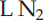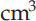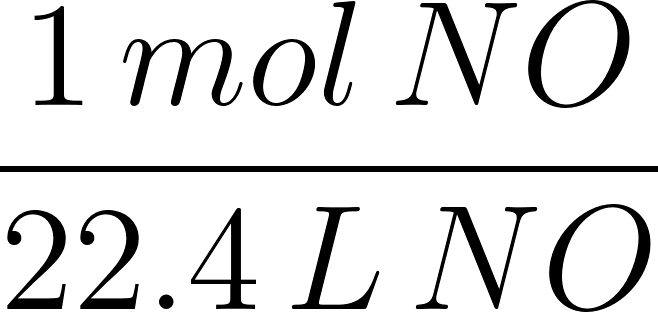×
Get Full Access to Introductory Chemistry - 5 Edition - Chapter 11 - Problem 88p
Get Full Access to Introductory Chemistry - 5 Edition - Chapter 11 - Problem 88p

×

# Calculate the mass of each gas sample at STP.(a) 5.82 L NO(b) 0.324 LN2(c) 139 cm3 ArISBN: 9780321910295 34

## Solution for problem 88P Chapter 11

Introductory Chemistry | 5th Edition

• Textbook Solutions
• 2901 Step-by-step solutions solved by professors and subject experts
• Get 24/7 help from StudySoup virtual teaching assistantsIntroductory Chemistry | 5th Edition

4 5 1 331 Reviews
17
4
Problem 88P

PROBLEM 88P

Calculate the mass of each gas sample at STP.

(a) 5.82 L NO

(b) 0.324 LN2

(c) 139 cm3 Ar

Step-by-Step Solution:

Problem 88P

Calculate the mass of each gas sample at STP.

(a) 5.82 L NO

(b) 0.324(c) 139Ar

Step by Step Solution

Step 1 of 3

(a)

At STP 22.4L contains one mole of molecules.The conversion factor will be;From the given,

The molecular mass of  NO = 30.01 g/molVolume of NO = 5.82L.

Let’s calculate the mass of 5.82 L NO.Therefore, the mass of 5.82 L NO gas at STP is 7.80 g.

Step 2 of 3

Step 3 of 3

##### ISBN: 9780321910295

Unlock Textbook Solution